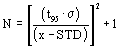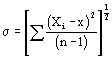# Title 40

## SECTION 1051.310

### 1051.310 How must I select vehicles or engines for production-line testing

§ 1051.310 How must I select vehicles or engines for production-line testing?

(a) Test engines from each engine family as described in this section based on test periods, as follows:

(1) For engine families with projected U.S.-directed production volume of at least 1,600, the test periods are consecutive quarters (3 months). However, if your annual production period is less than 12 months long, you may take the following alternative approach to define quarterly test periods:

(i) If your annual production period is 120 days or less, the whole model year constitutes a single test period.

(ii) If your annual production period is 121 to 210 days, divide the annual production period evenly into two test periods.

(iii) If your annual production period is 211 to 300 days, divide the annual production period evenly into three test periods.

(iv) If your annual production period is 301 days or longer, divide the annual production period evenly into four test periods.

(2) For engine families with projected U.S.-directed production volume below 1,600, the whole model year constitutes a single test period.

(b) Early in each test period, randomly select and test an engine from the end of the assembly line for each engine family.

(1) In the first test period for newly certified engines, randomly select and test one more engine. Then, calculate the required sample size for the model year as described in paragraph (c) of this section.

(2) In later test periods of the same model year, combine the new test result with all previous testing in the model year. Then, calculate the required sample size for the model year as described in paragraph (c) of this section.

(3) In the first test period for engine families relying on previously submitted test data, combine the new test result with the last test result from the previous model year. Then, calculate the required sample size for the model year as described in paragraph (c) of this section. Use the last test result from the previous model year only for this first calculation. For all subsequent calculations, use only results from the current model year.

(c) Calculate the required sample size for each engine family. Separately calculate this figure for HC, NOX (or HC + NOX), and CO. The required sample size is the greater of these calculated values. Use the following equation:Where: N = Required sample size for the model year. t95 = 95% confidence coefficient, which depends on the number of tests completed, n, as specified in the table in paragraph (c)(1) of this section. It defines 95% confidence intervals for a one-tail distribution. σ = Test sample standard deviation (see paragraph (c)(2) of this section). x = Mean of emission test results of the sample. STD = Emission standard (or family emission limit, if applicable).

(1) Determine the 95% confidence coefficient, t95, from the following table:

n t95 n t95 n t95
2 6.31 12 1.80 22 1.72
3 2.92 13 1.78 23 1.72
4 2.35 14 1.77 24 1.71
5 2.13 15 1.76 25 1.71
6 2.02 16 1.75 26 1.71
7 1.94 17 1.75 27 1.71
8 1.90 18 1.74 28 1.70
9 1.86 19 1.73 29 1.70
10 1.83 20 1.73 30 + 1.70
11 1.81 21 1.72

(2) Calculate the standard deviation, σ, for the test sample using the following formula:Where: Xi = Emission test result for an individual vehicle or engine. n = The number of tests completed in an engine family.

(d) Use final deteriorated test results to calculate the variables in the equations in paragraph (c) of this section (see § 1051.315(a)).

(e) After each new test, recalculate the required sample size using the updated mean values, standard deviations, and the appropriate 95-percent confidence coefficient.

(f) Distribute the remaining tests evenly throughout the rest of the year. You may need to adjust your schedule for selecting vehicles or engines if the required sample size changes. If your scheduled quarterly testing for the remainder of the model year is sufficient to meet the calculated sample size, you may wait until the next quarter to do additional testing. Continue to randomly select vehicles or engines from each engine family.

(g) Continue testing until one of the following things happens:

(1) After completing the minimum number of tests required in paragraph (b) of this section, the number of tests completed in an engine family, n, is greater than the required sample size, N, and the sample mean, x, is less than or equal to the emission standard. For example, if N = 5.1 after the fifth test, the sample-size calculation does not allow you to stop testing.

(2) The engine family does not comply according to § 1051.315.

(3) You test 30 vehicles or engines from the engine family.

(4) You test one percent of your projected annual U.S.-directed production volume for the engine family, rounded to the nearest whole number. Do not count a vehicle or engine under this paragraph (g)(4) if it fails to meet an applicable emission standard.

(5) You choose to declare that the engine family does not comply with the requirements of this subpart.

(h) If the sample-size calculation allows you to stop testing for one pollutant but not another, you must continue measuring emission levels of all pollutants for any additional tests required under this section. However, you need not continue making the calculations specified in this subpart for the pollutant for which testing is not required. This paragraph (h) does not affect the number of tests required under this section, the required calculations in § 1051.315, or the remedial steps required under § 1051.320.

(i) You may elect to test more randomly chosen vehicles or engines than we require under this section. Include these vehicles or engines in the sample-size calculations.

[67 FR 68347, Nov. 8, 2002, as amended at 70 FR 40498, July 13, 2005; 73 FR 59251, Oct. 8, 2008]